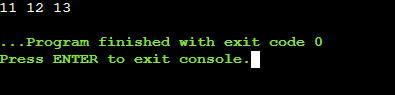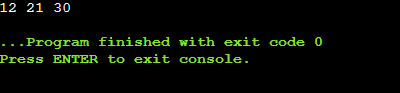# How to Initialize a Vector in C++?

Do you know “How to Initialize Vector Cpp“? Have you ever thought what is the process to initialize Vector Cpp? C++ is an important language to learn if you want to have a successful career in the programming field. Initializing a vector in C++ is one of the important concepts. Let us gain some knowledge about the process and ways to initialize it.

Still, if you have any issues while solving your C++ assignment then you can get C++ assignment help online by hiring the proficient experts at CodingZap.

But before we start knowing about the Vector in C++, let us try to find the necessity of it from an example of our daily life.

##### Real-Life Example :

Suppose, you want to build up a bookshelf for yourself. This will help to store your storybooks & comics in a good manner. And you can able to develop it by yourself. You don’t want to take the help of any carpenter. So, you bring some materials from the market & start developing it.

Now, after developing it, you start putting books into it. After some time you realize that you have made the bookshelf a smaller one. There is not enough space to store more books. What will you do in such cases?

Well, you don’t have any other option & you have to manage to store books in it. So basically, you have made a complete mess by developing the bookshelf. As you don’t aware of the size of books, you have developed a small one.

Now, suppose this same problem is happening to your program. The input data is a lot more than the storing space-like array. So, what will you do in such situations? In such cases, you have to initialize Vector Cpp. This will help to come out of the such situation.

Now, let us first know the definition of Vector briefly to understand the topic.

### What Is Vector? Get To Know

Vector is not the same thing that we have learned in our physics classes. Also, it is not the same thing that spread diseases among human. Vector is a special component in programming languages. It is mainly associated with Object-Oriented Programming Language. It can be seen in the C++ programming language. Vector helps to store data in itself. It can only able to store similar kinds of data to it. This means all the integers or the value of the character can be stored in one single vector.

It seems like the array. But this is different from the array in some mean. It only differs from the size perspective. The size of the array can’t be changed. Once it is declared, it will be fixed. But the size of the vector can be changed. Sometimes a large number of data can be stored there. It helps to develop the code without thinking about the size of the memory.

There are many other definitions which are important to know while learning C++, one of them in Memory Leaks. So, if you want to know about this then you can read our article here.

In the C programming language, we sued dynamic programming to store such type of data. We called them Dynamic Memory Allocation. Here, instead of that, we are using vectors for the same purpose. For getting a large number of the same kind of data, we need to initialize Vector Cpp.

If you’re curious to know about Dynamic Memory Allocation, then you can check out our article on “Dynamic Memory Allocation In C

## What Are The Methods To Initialize Vector Cpp? Read Below

There are a lot of methods present to initialize vector Cpp. Some of them can assume as the repetition of another method. But they are completely different from each other. Depending upon the use & importance, we can able to make a list of six methods. These methods can be used to initialize Vector Cpp.

1. By Using Push_Back() Method
2. By Using Size Of Vector
3. By Using Array
4. By Copying From Another Vector
5. By Using The Index Of Vector
6. By Using Fill() Method

Let us know about each & every method one by one briefly. But before that, we need to know the basic declaration of the Vector.Also if you want to know about the methods involved in creating C++ dictionary then read our article on “How To Create C++ Dictionary?

### What Is The Basics Of Declaration Of Vector? Read Below

For declaring a Vector in C++, we have to first include one header file. This header file will help to use the Vector & other methods associated with the Vector in C++. This is the library header file. After adding the header file we need to declare the vector.

For declaring the vector, we need to follow one specific syntax. First, we need to write a vector. Then inside the <> symbol, we need to write the nature of the data. This means we are going to add the integer value of the character value to the vector. Then we have to provide the name of it. This is the simple declaration of the vector. Then according to the method, we can make changes there.

General Syntax: vector<data-type> name;

### How To Initialize Vector Cpp By Using Push_Back() Method?

Here, we have to first initialize the vector in the normal form. Then we have to take the help of the inbuilt method push_back(). This will help to add each & every piece of data to the Vector. Here, we can add many data without declaring the size of the vector. This method is very helpful.

General Syntax: vectorname. push_back(value)

Example:

```#include <iostream>

#include <vector>

using namespace std;

int main(){

vector<int> zap;  // Create Empty Vector

// Adding Values One By One

zap.push_back(1);

zap.push_back(2);

for (int x : zap)

cout << x << " ";  // Printing Values

return 0;}```

Let us try to find out the output of the above code. This will help to know the process to initialize Vector Cpp.

Output:### How To Initialize Vector Cpp By Using Size Of Vector?

Here, we need to use the default size method of the Vector. We have to provide the size along with the value. This will help to add value that much times to it. In this way, we can able to add some data to Vector easily.

General Syntax: vector<data-type> vectorname(size, value);

Example:

```#include <iostream>

#include <vector>

using namespace std;

int main(){

vector<int> zap(5, 2);  // Vector Of Size 2 With All Values As 5

for (int x : zap)

cout << x << " ";  // Printing Values

return 0;}

```

Let us try to find out the output of the above code. This will help to know the process to initialize Vector Cpp.

Output:### How To Initialize Vector Cpp By Using Array?

Here, we have to initialize the vector as the array. As the array, we have to provide values to it. In this way also, we can able to insert values into it.

General Syntax: vector<data-type> vectorname{value1, value2….};

Example:

```#include <iostream> #include <vector>

using namespace std;

int main(){

vector<int> zap{100, 200, 300};  // Initializing Like Array

for (int x : zap)

cout << x << " ";  // Printing Values

return 0;}

```

Let us try to find out the output of the above code. This will help to know the process to initialize Vector Cpp.

Output:### How To Initialize Vector Cpp By Copying From Another Vector?

Here, first, we have to initialize one vector. We have taken one array to initialize the first vector. Then from the first vector, we have to make the second vector by copying it. For that purpose, we need to like type case it. The new vector will be placed at the outside of the brackets. This will help to copy it.

General Syntax: vector<data-type> vectorname2(vectorname1);

Example:

```#include <iostream> #include <vector>

using namespace std;

int main(){

vector<int> zap{11, 12, 13};  // Initializing Like Array

vector<int> codingzap(zap);  // Copying Vector

for (int x : codingzap)

cout << x << " ";  // Printing Values

return 0;}

```

Let us try to find out the output of the above code. This will help to know the process to initialize Vector Cpp.

Output:### How To Initialize Vector Cpp By Using Index Of Vector?

By using the index of the vector, we can provide the values to it. First, we need to initialize the vector with size. Then we have to provide the values to it one by one using the index of the vector.

Example:

```#include <iostream>  #include <vector>

using namespace std;

void main(){

vector<int> zap(3);  // Initializing Vector With Size

zap=12; zap=21; zap=30;  // Providing Value With Index

for (int x : zap)

cout << x << " ";}  // Printing Values```

Let us try to find out the output of the above code. This will help to know the process to initialize Vector Cpp.

Output:### How To Initialize Vector Cpp By Using Fill() Method?

In this case, also, we need to declare the vector with the size. Then we have to use the fill() method. this method will also help to add the same value to the vector in the repetitive format. There are two arguments. One is for starting of the vector & another is for the ending of the method. Also, we have to provide the value there.

General Syntax: fill(vectorname.begin(),vectorname.end(),value);

Example:

```#include <iostream> #include <vector>

using namespace std;

int main(){

vector<int> zap(3);  // Initializing Vector With Size

fill(zap.begin(),zap.end(),3);  // Using Fill Method

for (int x : zap)

cout << x << " ";  // Printing Values

return 0;}

```

Let us try to find out the output of the above code. This will help to know the process to initialize Vector Cpp.

Output:## Conclusion:

As we saw initialize Vector Cpp is a very important topic.

We need to first clear the basics of the C++ programming language. This will help a lot to understand the topic in a better manner.

The Vector in C++ will help you a lot in the future. This is the key to remove out the problems related to the space problem of data input. We have to practice it in a good manner.

Still having issues understanding, It is advisable to clear the basics of the C++ programming language, you can always hire a programming tutor from CodingZap . It will be easy for us to gain knowledge about this topic in an easy & proper manner.

So, hope you have liked this piece of article. Share your thoughts in the comments section and let us know if we can improve more.